# How to generate only random even or odd numbers in Excel?

Random data is a type of data generated randomly. For this tutorial the focus is to generate random even and odd numbers. Random data can be used to many purpose such as creating a sample data set, or to evaluate results on the based on the dataset. In this tutorial, the main focus is to generate the odd and even number between the specified data, by using the predefined available excel methods, and custom formulas. This tutorial contain two example first example will allow user to generate random odd number and latter one allows user to generate random even number.

## Example 1 to generate random odd number

Step 1

To understand the process of generating random number. Let’s create some sample excel sheet with starting and end numbers. Both these numbers can be used as a range and the new generated random data should lie between these two numbers and should be an odd number.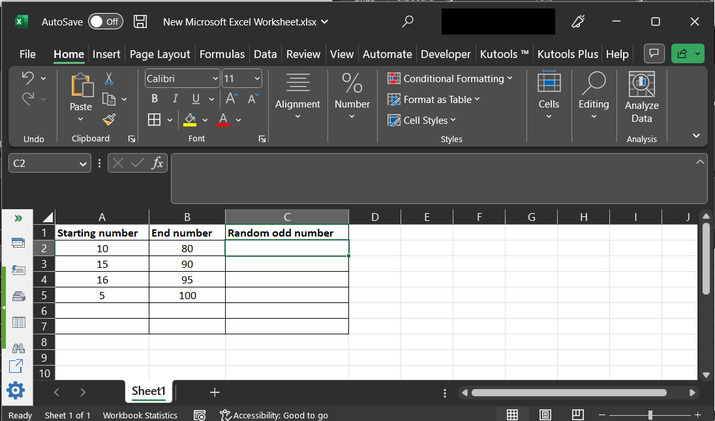Step 2

Go to the C2 cell, and type the formula “=ODD(RANDBETWEEN(A2,B2))”.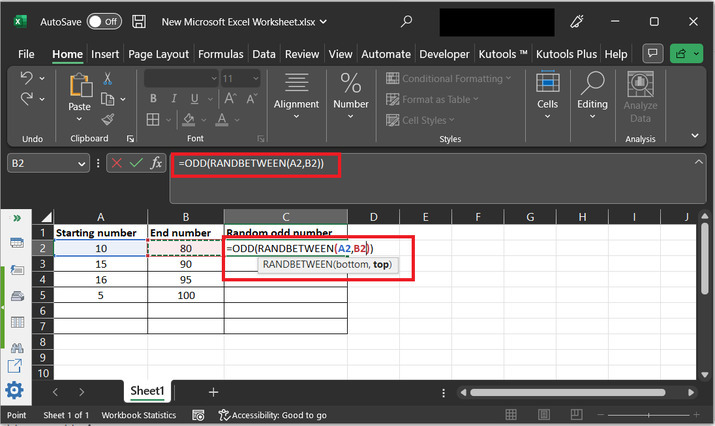Explanation for ODD and RANDBETWEEN method

Both the above-mentioned methods are predefined and all already present in the MS excel. ODD() method allow user to generate odd values, such as 1, 3, 5, and many others.

While, RANDBETWEEN() method allow user to create a random data value from the specified range. This method can read data either by passing the values directly or by passing the cell references ( as used in this tutorial).

Step 3

Press Enter key and the result for first row will be generated, as depicted below: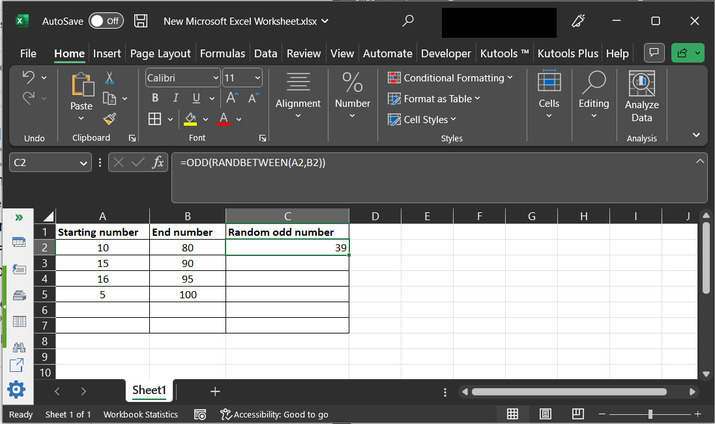Step 4

After that click on the right corner of the C2 cell. A plus sign will be displayed there, simply drag that plus sign to 5 th row. This will copy the same formula, with different row values. The final obtained results are shown below: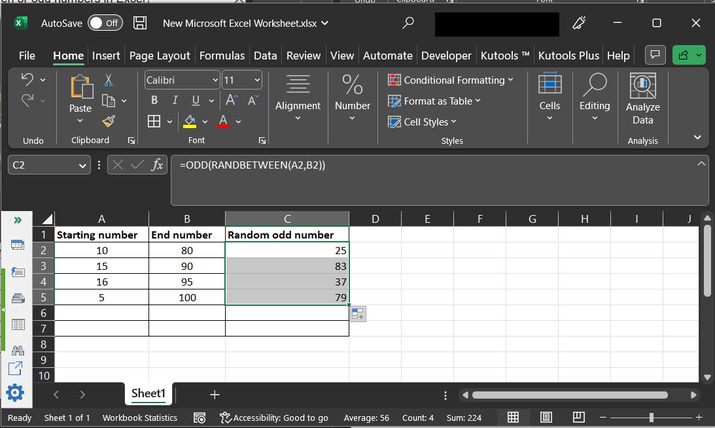## Example 2 to generate random even number

Step 1

Again, consider same worksheet, this time simply replace the C column header data from “odd” to “even”. Both these numbers can be used as a range and the new generated random data should lie between these two numbers and should be even numbers.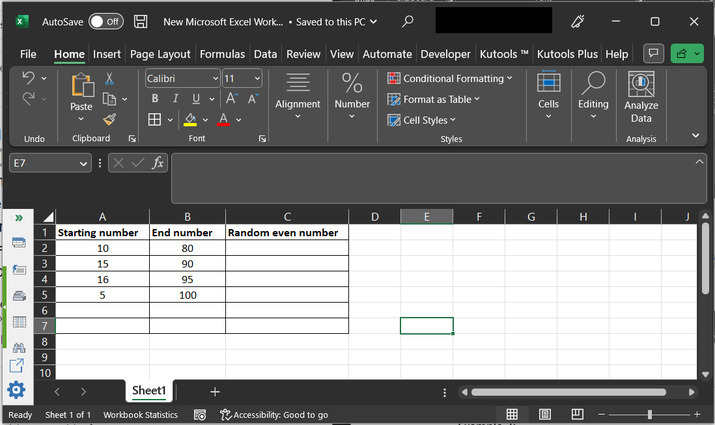Step 2

Go to the C2 cell, and type the formula “=EVEN(RANDBETWEEN(A2,B2))”.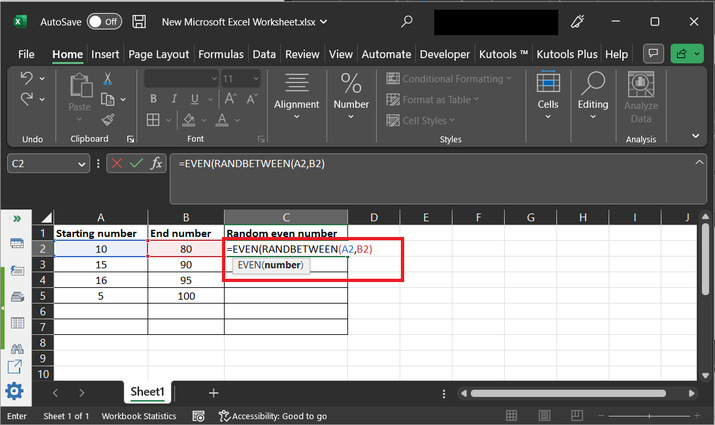EVEN() method will allow user to generate even numeric values. Examples of even numeric values are 2, 4, 6, 8, and many other.

Step 3

Press “Enter key” and the result for first row will be generated, as depicted below: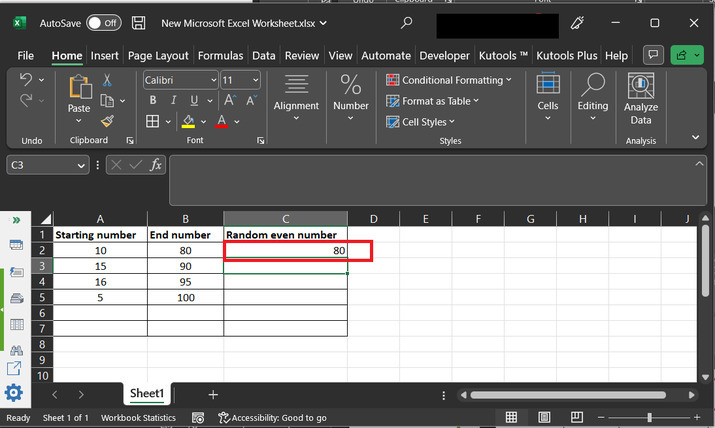Step 4

After that click on the right corner of the C2 cell. A plus sign will be displayed there, simply drag that plus sign to 5 th row. This will copy the same formula, with different row values. The final obtained results are shown below: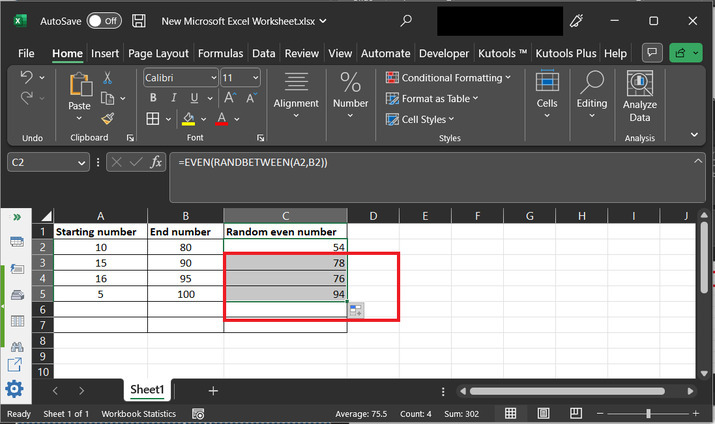## Conclusion

This tutorial provides two simple examples to generate random even and odd numeric values. Both the methods use the predefined excel function. EVEN() method will assure that the random number generated by the RANDBETWEEN() method is even and similarly, ODD() method will allow user to generate odd numbers.

Updated on: 22-Aug-2023

98 Views